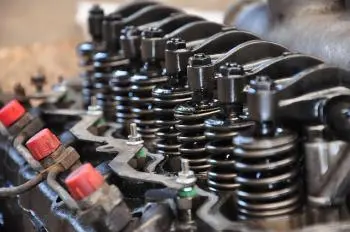# Hooke's Law: Formula, Applications, Examples and Exercises ResultsHooke's law is a fundamental principle in physics that describes the elastic behavior of materials, formulated by the English scientist Robert Hooke in the 17th century.

The law states that the force required to deform an elastic material is directly proportional to the magnitude of the deformation, as long as the elastic limit of the material is not exceeded.

Hooke's law is a valid approximation only in the elastic range of materials, that is, when the deformation does not exceed the elastic limit. If the strain is excessive, the material may enter the plastic region, where the proportionality between force and strain is not met.

Hooke's law of elasticity is applied in a wide variety of situations, from the study of springs and elastics to the analysis of deformable structures and materials in engineering and physical sciences.

## Hooke's Law Formula

Mathematically, Hooke's law of elasticity is expressed by the formula:

F=-kx

Where:

• F is the force exerted on the material.

• k is the constant of elasticity (also known as elastic constant or spring constant), which depends on the properties of the material.

• x is the deformation experienced by the material.

The force F has a direction opposite to the deformation x, which means that the material will tend to return to its original shape when the external force that deforms it is suspended.

## Hooke's Law Applications

Hooke's law has a wide range of applications in various fields of science and engineering. Some of the most common uses are:

1. Springs and elastic systems: this law is fundamental in the study and design of systems that involve springs, such as automobile suspensions, suspension systems in buildings, musical instruments, damping systems, among others. It allows predicting how these elastic systems will behave when faced with an applied force and how they will deform.

2. Elastic Materials: In the field of materials engineering, Hooke's law is used to understand and predict the elastic behavior of various materials, such as metals, polymers, and composite materials. It aids in the design of structures that need to have specific elastic properties, such as bridges, buildings, and medical devices.

3. Structural analysis: Hooke's law is applied in the analysis of structures and beams to calculate the strains and stresses resulting from applied loads. This is critical in civil and mechanical engineering to ensure the safety and performance of structures.

4. Medicine and biomechanics: In the field of medicine and biomechanics, it is used to study the behavior of biological tissues, such as bones, tendons, ligaments, and muscle tissue. It helps to understand how these tissues deform and recover in response to external forces and is useful in the design of prosthetics, implants, and medical devices.

5. Measuring instruments: Hooke's law is used in various measuring instruments, such as extensometers and strain gauges, which are used to measure strains and stresses in materials. This is especially useful in material testing and strength testing.

## Examples in Day to Day

Here are some everyday examples where Hooke's Law works:

1. Door springs: When a force is applied to open the door, the spring stretches and when it is released, the spring contracts, returning the door to its original position.

2. Trampolines: Trampolines used in recreational activities also make use of Hooke's law. When a person jumps on the trampoline, the elastic material deforms and stretches depending on the applied force. By releasing the force, the trampoline returns to its original shape, propelling the person upwards.

3. Car Suspension: Suspension systems in cars use springs and shock absorbers that support the weight of the vehicle and compress or stretch based on bumps in the road. This allows for a smoother ride and prevents impacts from being transmitted directly to the car's chassis.

4. String musical instruments: Instruments such as the guitar, violin or piano use strings that follow Hooke's law. By pressing or rubbing the strings, they deform and vibrate, generating musical sounds. The applied tension and the deformation of the strings follow the relationship established by Hooke's law.

5. Spring Compression in Ballpoint Pens: Ballpoint pens have a spring in their internal mechanism. Pressing the button or end of the pen compresses the spring following Hooke's law. When you release the button, the spring expands, pushing the tip of the pen out.

## Solved Exercises of the Law of Elasticity

Here are some solved exercises:

### Exercise 1

A spring has a constant of elasticity of 50 N/m. If a force of 20 N is applied to the spring, what will be the deformation experienced by the spring?

Solution : Using Hooke's law formula: F = -kx Where F is the applied force, k is the constant of elasticity and x is the deformation.

Rearranging the formula to solve for x: x = -F / k

Substituting the values: x = -20 N / (50 N/m) x = -0.4 m

The deformation experienced by the spring is -0.4 meters. The negative sign indicates that the deformation is in the opposite direction to the applied force.

### Exercise 2

A spring has a deflection of 0.2 m when a force of 30 N is applied to it. Determine the constant of elasticity of the spring.

Solution : Using Hooke's law formula: F = -kx Where F is the applied force, k is the constant of elasticity and x is the deformation.

Rearranging the formula to solve for k: k = -F / x

Substituting the values: k = -30 N / 0.2 mk = -150 N/m

The spring constant of the spring is -150 N/m. The negative sign indicates that the spring behaves as a restoring force, that is, it opposes the deformation.

Author:

Published: July 13, 2023
Last review: July 13, 2023